Next: Elliptic expansions Up: Useful mathematics Previous: Curvilinear coordinates

# Conic sections

The ellipse, the parabola, and the hyperbola are collectively known as conic sections, because these three types of curve can be obtained by taking various different plane sections of a right cone.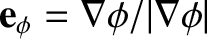An ellipse, centered on the origin, of major radiusand minor radius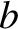, which are aligned along the- and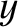-axes, respectively (see Figure A.4), satisfies the following well-known equation: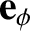(A.101)

where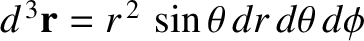.

Likewise, a parabola which is aligned along the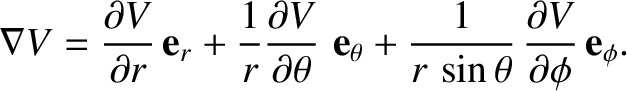-axis, and passes through the origin (see Figure A.5), satisfies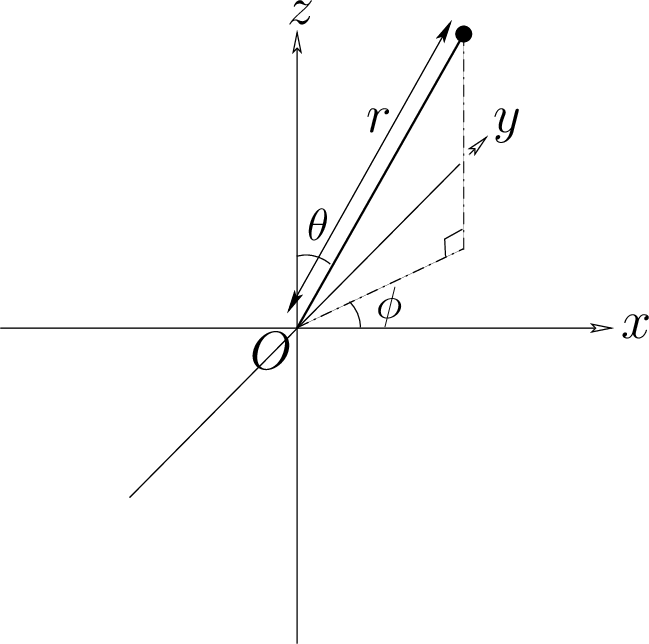(A.102)

where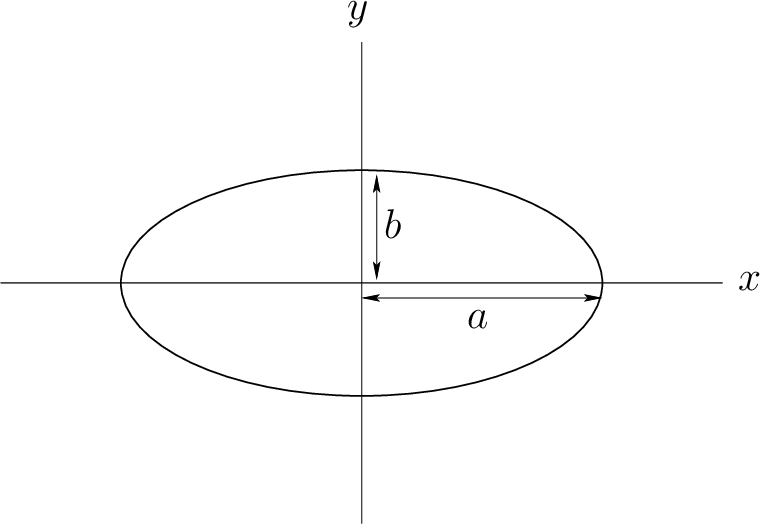.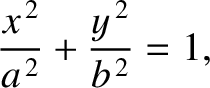Finally, a hyperbola which is aligned along the-axis, and whose asymptotes intersect at the origin (see Figure A.6), satisfies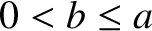(A.103)

where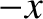. Here,is the distance of closest approach to the origin. The asymptotes subtend an angle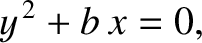with the-axis.

It is not obvious, from the preceding formulae, what the ellipse, the parabola, and the hyperbola have in common. It turns out, in fact, that these three curves can all be represented as the locus of a movable point whose distance from a fixed point is in a constant ratio to its perpendicular distance to some fixed straight line. Let the fixed point--which is termed the focus--lie at the origin, and let the fixed line--which is termed the directrix--correspond to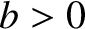(with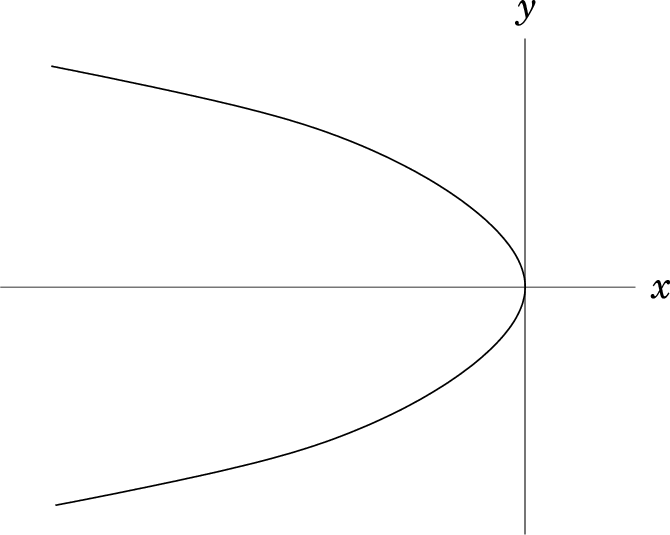). Thus, the distance of a general point (,) (which lies to the left of the directrix) from the focus is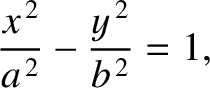, whereas the perpendicular distance of the point from the directrix is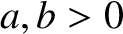. (See Figure A.7.) In polar coordinates,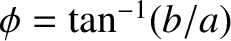and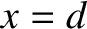. Hence, the locus of a point for which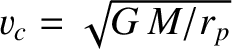and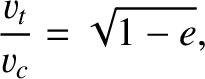are in a fixed ratio satisfies the following equation: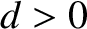(A.104)

where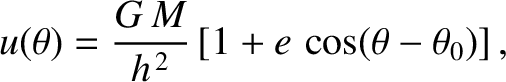is a constant. When expressed in terms of polar coordinates, the preceding equation can be rearranged to give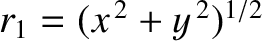(A.105)

where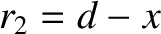.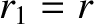When written in terms of Cartesian coordinates, Equation (A.104) can be rearranged to give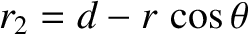(A.106)

for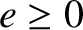. Here,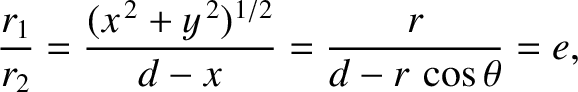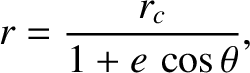(A.107)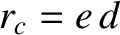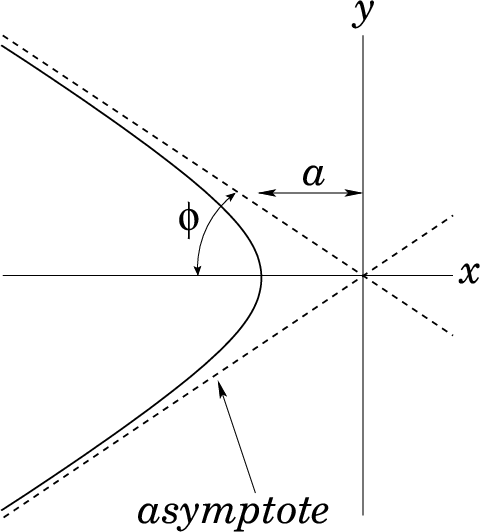(A.108) and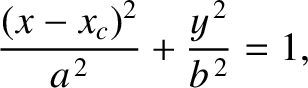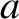(A.109)

Equation (A.106) can be recognized as the equation of an ellipse whose center lies at (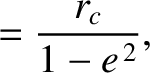, 0 ), and whose major and minor radii,and, are aligned along the- and-axes, respectively [see Equation (A.101)]. Note, incidentally, that an ellipse actually possesses two focii located on the major axis (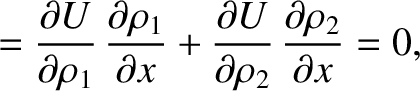) a distanceon either side of the geometric center (i.e., at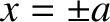and). Likewise, an ellipse possesses two directrices located at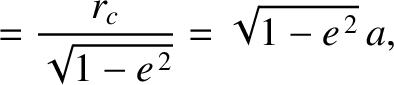.

When again written in terms of Cartesian coordinates, Equation (A.104) can be rearranged to give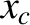(A.110)

for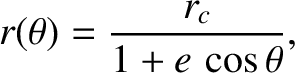. Here,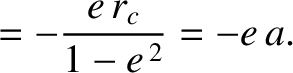. This is the equation of a parabola that passes through the point (, 0 ), and which is aligned along the-direction [see Equation (A.102)].Finally, when written in terms of Cartesian coordinates, Equation (A.104) can be rearranged to give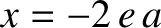(A.111)

for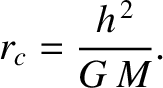. Here,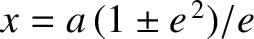(A.112)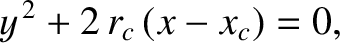(A.113) and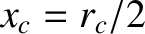(A.114)

Equation (A.111) can be recognized as the equation of a hyperbola whose asymptotes intersect at (, 0 ), and which is aligned along the-direction. The asymptotes subtend an angle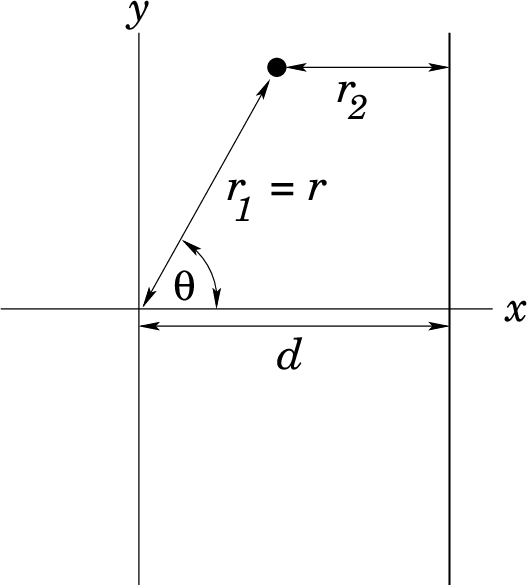(A.115)

with the-axis [see Equation (A.103)].

In conclusion, Equation (A.105) is the polar equation of a general conic section that is confocal with the origin (i.e., the origin lies at a focus). For, the conic section is an ellipse. For, the conic section is a parabola. Finally, for, the conic section is a hyperbola.Next: Elliptic expansions Up: Useful mathematics Previous: Curvilinear coordinates
Richard Fitzpatrick 2016-03-31